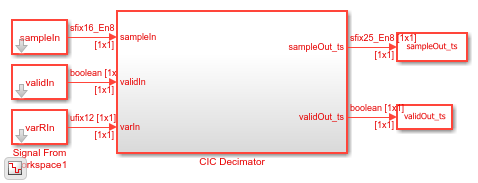# Implement CIC Decimator Filter for HDL

This example shows how to use a CIC Decimator block to filter and downsample data. This block supports scalar and vector inputs. To work with scalar and vector inputs separately, this example uses two Simulink® models. You can generate HDL code from the subsystems in these Simulink models.

### Set Up Input Data Parameters

Set up these workspace variables for the models to use. These variables configure the CIC Decimator block inside of them. This block supports fixed and variable decimation rates for scalar inputs and only a fixed decimation rate for vector inputs. The example runs the `HDLCICDecimatorModel.slx` model when you set the `scalar` value to `true` and runs the `HDLCICDecimatorModelVectorSupport.slx` model when you set the `scalar` value to `false`. For scalar inputs, choose a range for the input `varRValue` values and set the decimation factor value, `R`, to the maximum expected decimation factor. For vector inputs, the input data must be a column vector of size 1 to 64. `R` must be an integer multiple of input frame size.

```R = 8; % Decimation factor M = 1; % Differential delay N = 3; % Number of sections scalar = true; % true for scalar; false for vector if scalar varRValue = [4,R]; vecSize = 1; modelname = "HDLCICDecimatorModel"; else varRValue = R; %#ok fac = (factor(R)); vecSize = fac(randi(length(fac),1,1)); modelname = "HDLCICDecimatorModelVectorSupport"; end numFrames = length(varRValue); dataSamples = cell(1,numFrames); varRtemp = cell(1,numFrames); cicFcnOutput = []; WL = 0; % Word length FL = 0; % Fraction length ```

### Generate Reference Output from `dsp.CICDecimator` System Object

Generate frames of random input samples and provide them as input to the `dsp.CICDecimator` System object™. The output generated from this System object is used as a reference data for comparison. This System object does not support variable decimation rates, so you must create and release this object for any change in the decimation factor value.

```for i = 1:numFrames framesize = varRValue(i)*randi([5 20],1,1); dataSamples{i} = fi(randn(vecSize,framesize),1,16,8); varRtemp{i} = fi(varRValue(i)*ones(framesize,1),0,12,0); obj = dsp.CICDecimator("DifferentialDelay",M,"NumSections",N, ... "DecimationFactor",varRValue(i)); cicOut = step(obj,dataSamples{i}(:)).'; WL = max([WL,cicOut.WordLength]); FL = max([FL,cicOut.FractionLength]); cicFcnOutput = [fi(cicFcnOutput,1,WL,FL),cicOut]; release(obj); end ```

### Convert Input to Stream of Samples and Import them to Simulink Model

Generate a stream of samples by converting frames to samples. Provide those samples (`sampleIn`) and the valid signal (`validIn`) as inputs to the Simulink model. The latency of the block for scalar and vector inputs is calculated based on the type of input and the number of sections, `N`. For more information, see Latency. To flush remaining data, run the model by inserting the required number of idle cycles after each frame using the `idlecyclesbetweenframes` value.

```idlecyclesbetweensamples = 0; idlecyclesbetweenframes = floor((vecSize-1)*(N/vecSize))+ 1 + N + ... (2+(vecSize+1)*N) + 9; sampleIn = []; validIn = []; varRIn = []; len = 0; for ij = 1:numFrames dataInFrame = dataSamples{ij}; if scalar len = length(dataInFrame); else len = size(dataInFrame,2); %#ok end data = []; valid=[]; varR = []; for ii = 1:len data = [data dataInFrame(:,ii) ... zeros(vecSize,idlecyclesbetweensamples)]; %#ok valid = [valid true(1,1) ... false(1,idlecyclesbetweensamples)]; %#ok varR = [varR varRtemp{ij}(ii) ... zeros(1,idlecyclesbetweensamples)]; %#ok end sampleIn = cast([sampleIn,data, ... zeros(vecSize,idlecyclesbetweenframes)],"like",dataInFrame); validIn = logical([validIn,valid,zeros(1,idlecyclesbetweenframes)]); varRIn = fi([varRIn,varR,zeros(1,idlecyclesbetweenframes)],0,12,0); end sampletime = 1; simTime = length(validIn); ```

Run the model. Running the model imports the input signal variables from the MATLAB® workspace to the CIC Decimator block in the model.

```open_system(modelname); sim(modelname); ```### Compare Simulink Block Output with MATLAB System Object Output

Capture the output of the Simulink block. Compare that output with the output of the `dsp.CICDecimator` System object.

```sampleOut = squeeze(sampleOut_ts.Data).'; validOut = squeeze(validOut_ts.Data); cicOutput = sampleOut(validOut); fprintf('\nCIC Decimator\n'); difference = (abs(cicOutput-cicFcnOutput(1:length(cicOutput)))>0); fprintf(['\nTotal number of samples that differed between Simulink block ' ... 'output and MATLAB System object output: %d \n'],sum(difference)); ```
```CIC Decimator Total number of samples that differed between Simulink block output and MATLAB System object output: 0 ```